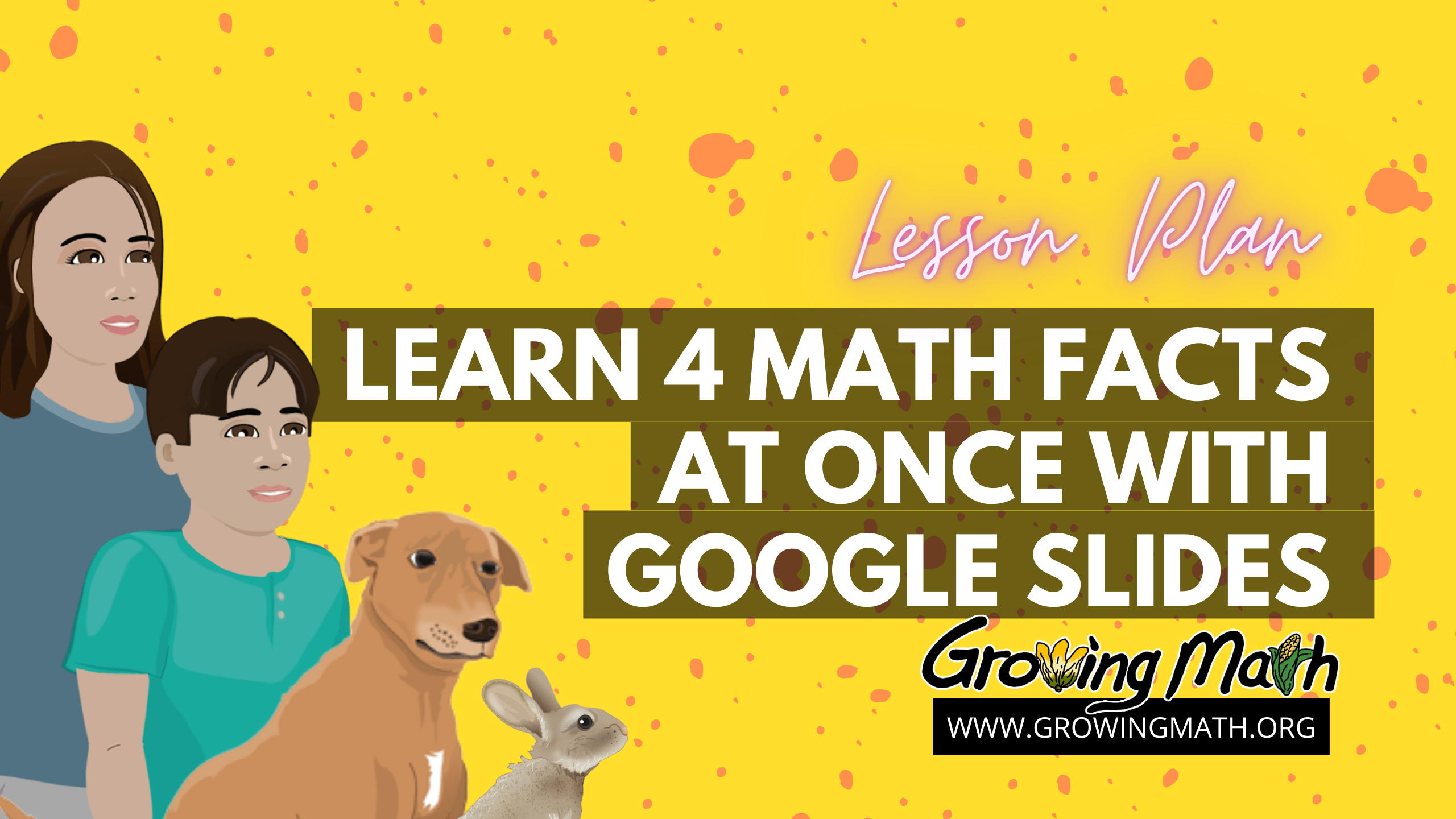# Learn 4 Math Facts at Once with Google Slides

## Standard

CCSS.MATH.CONTENT.3.OA.A.1 Interpret products of whole numbers, e.g., interpret 5 × 7 as the total number of objects in 5 groups of 7 objects each.

CCSS.MATH.CONTENT.3.OA.A.2 Interpret whole-number quotients of whole numbers, e.g., interpret 56 ÷ 8 as the number of objects in each share when 56 objects are partitioned equally into 8 shares.

CCSS.MATH.CONTENT.4.NBT.B.4
Fluently add and subtract multi-digit whole numbers using the standard algorithm.

45-60 minutes

## Summary

Start with a video as an ice breaker. Then, students read or listen to a presentation explaining how each math fact is actually four. Students complete an activity where they create their own math facts slides. A recommended video explains features of Google slides. Students complete the lesson playing Making Camp Dakota, solving word problems using division.

## Listen to/ Read a presentation on four math facts at a time

This presentation explains how one math fact is actually four because 7 x 6 = 42 means that 42 ÷ 7 = 6 and also that 6 x 7 = 42 and 42 ÷ 6 = 7. As an added bonus, it includes some ranch vocabulary like cattle, steer, heifer and bull.

After the teacher has given the first part of the presentation, students are challenged to first show how a given math fact is actually four math facts, using steers. Next, students create their own examples. The easiest way to do this is through assignment in Google classroom or other system, including email, giving students their own copies of the slides to modify.

### Recommended Video: Math Facts with Google Slides

If your students are unsure how to copy and paste, how to select multiple elements at a time, rotate objects or insert a duplicate slide, it is all in this video. Why not watch the video first? Because students often pay more attention once they realize they have questions that can be assigned by the assigned video.